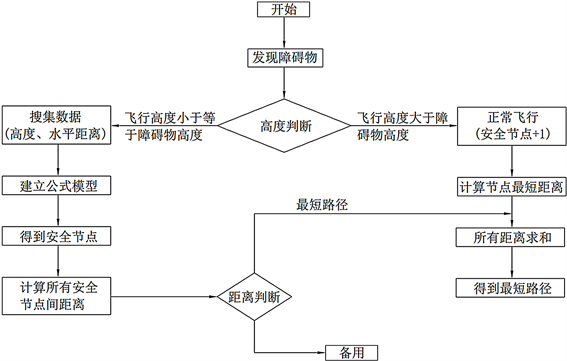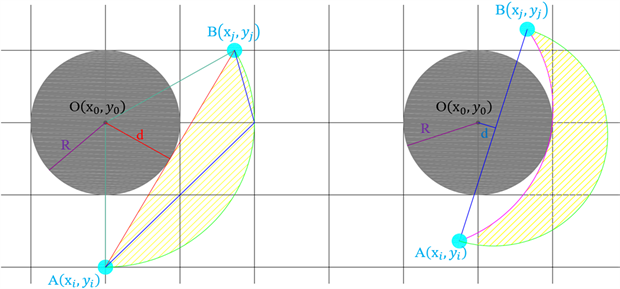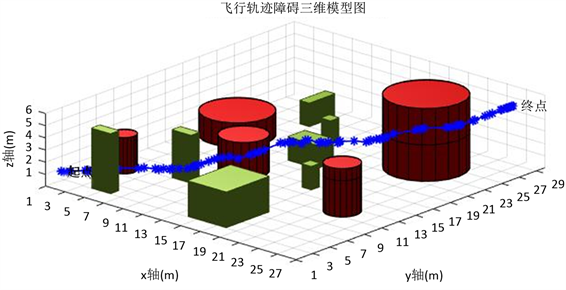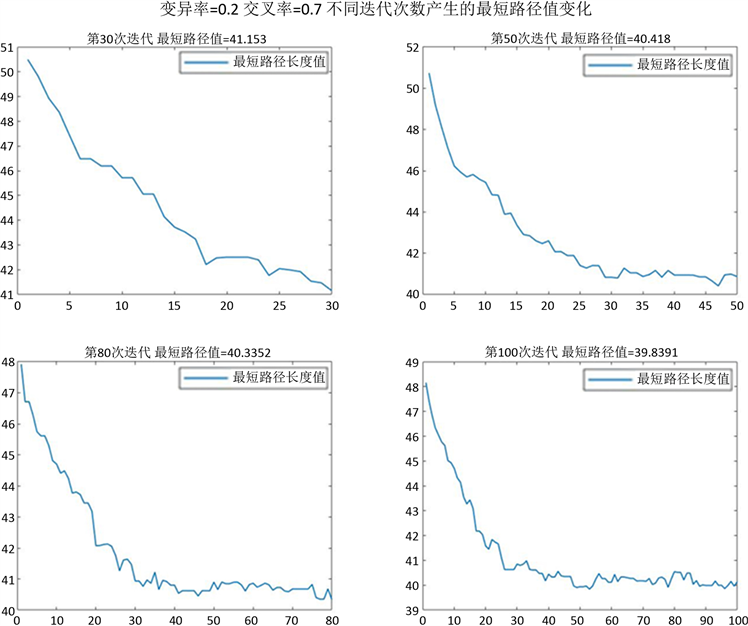#### 期刊菜单

Intelligent UAV Path Planning in Emergency Rescue
DOI: 10.12677/JAST.2021.92005, PDF, HTML, XML, 下载: 194  浏览: 442  科研立项经费支持

Abstract: This paper is proposed to make the unmanned aerial vehicle (UAV) in a real environment safe obstacle avoidance more safely and effectively, based on the improved adaptive genetic algorithm of unmanned aerial vehicle (UAV) obstacle avoidance path planning model, using the matlab simulate the 3D model of the obstacles and scope of space, after using a genetic algorithm for unmanned aerial vehicle (UAV) completed in the 3D environment is safe and effective path planning, Through the simulation under different shapes of obstacles, the simulation results show that the improved obstacle avoidance path planning model can quickly find a shorter and safer path, and achieve the purpose that the UAV can quickly implement rescue in emergency situations.

1. 引言

2. 地图预处理Figure 1. Three-dimensional model diagram of flight trajectory obstacle

3. 算法设计流程Figure 2. Design flow chart

4. 满足无人机安全飞行公式建模

$d\left(i,j\right)=\sqrt{{\left({x}_{i}-{x}_{j}\right)}^{2}+{\left({y}_{i}-{y}_{j}\right)}^{2}+{\left({z}_{i}-{z}_{j}\right)}^{2}}$ (1)

$D=\underset{i=1}{\overset{n}{\sum }}\underset{j=1}{\overset{m}{\sum }}\mathrm{min}\left(d\left(i,j\right)\right)+{l}_{偏航}$ (2)

$\left({x}_{i},{y}_{i},{z}_{i}\right)$$\left({x}_{j},{y}_{j},{z}_{j}\right)$ ——任意两安全节点的坐标

D——总路径长度

$\left({y}_{j}-{y}_{i}\right)x+\left({x}_{i}-{x}_{j}\right)y+{x}_{j}{y}_{i}-{x}_{i}{y}_{j}=0$ (3)

$d=\frac{|A{x}_{0}+B{y}_{0}-C|}{\sqrt{{A}^{2}+{B}^{2}}}$ (4)

$\left\{\begin{array}{l}R>d\text{\hspace{0.17em}}\text{\hspace{0.17em}}\text{\hspace{0.17em}}\text{\hspace{0.17em}}障碍物与直线相交\\ R(a) 节点连线与障碍物相切 (b) 节点连线与障碍物相交

Figure 3. The position relationship between the connections between nodes and obstacles

5. 遗传算法规划

5.1. 遗传算法原理

5.2. 利用遗传算法模拟路径

$\text{fitness}=1/D$ (5)Figure 4. Flight trajectory diagram

5.3. 基于遗传算法路径分析Figure 5. Evolution process diagram of genetic algorithm

6. 结论

1) 利用matlab模拟出障碍物三维形状，将整个三维地图进行网格化处理，便于找出每个点的坐标，且将每个点坐标分开存储，便于实现点与点间的直接连接，提高算法的运行效率。

2) 在模型迭代次数方面，在进行路径搜索最优解过程中，本文发现，以最短路径值为判断标准的进化曲线，当迭代次数越小，其收敛速度越慢，进化曲线越不稳定，而迭代次数越多，规划模型则表现出了更为优越的稳定性和收敛速度，通过该方案可以有效提升无人机在灾害救援任务中的响应能力以及救援效率。

  吕明, 郑静晨, 陈金宏, 刘皓挺, 高云. 基于FPGA技术的灾害救援无人机图像采集与分析系统设计[J]. 医疗卫生装备, 2016, 37(8): 6-9.  于涛. 基于改进蚁群算法的三维无人机路径规划的研究与实现[D]: [硕士学位论文]. 重庆: 重庆大学, 2017.  祁永强, 李春阳, 刘晓婷. 基于人工水流思想的无人机三维路径规划[J]. 徐州工程学院学报(自然科学版), 2020, 35(4): 38-47.  郭一聪, 刘小雄, 章卫国, 杨跃. 基于改进势场法的无人机三维路径规划方法[J]. 西北工业大学学报, 2020, 38(5): 977-986.  吴亚雷. 基于改进的A~*算法在三维路径规划中的仿真应用[J]. 农业装备与车辆工程, 2019, 57(6): 50-54.  莫汉培, 陈秋良, 张子臻. 遗传算法求解电力设施选址问题[J]. 计算机技术与发展, 2016, 26(3): 197-201.  李迪, 黄德才. 基于免疫遗传算法的网格工作流服务选择[J]. 浙江工业大学学报, 2010, 38(6): 673-678.  冯现东. 无人机室内避障及场景三维重建技术的研究[D]: [硕士学位论文]. 青岛: 青岛科技大学, 2018.  张西忱. 无人机的避障技术及其应用[J]. 集成电路应用, 2017(2): 66-68.  明宇. 基于视觉的植保无人机避障研究[D]: [博士学位论文]. 沈阳: 沈阳理工大学, 2018.  吴正阳. 城市轨道交通网络客流分配理论与控制技术研究[D]: [硕士学位论文]. 成都: 西南交通大学, 2018.  卢永飞. 饱和多孔介质的参数反演分析[J]. 中国建材科技, 2015, 24(5): 3.  王银玲, 李华聪. 遗传算法优化的碳纤维复合材料声发射数据聚类分析[J]. 无损检测, 2019, 41(6): 1-5+37.